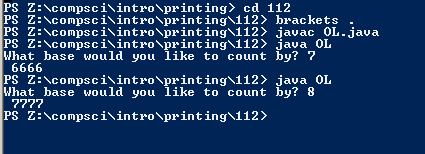# 112

## Code

```    ///Name: Joshua Bautista
///Period: 6
///Date: 6/4/16

import java.util.Scanner;

public class OL
{
public static void main( String[] args ) throws Exception
{
Scanner kb = new Scanner (System.in);

System.out.print("What base would you like to count by? ");
int n = kb.nextInt();

for ( int thous=0; thous < n; thous++ )

for ( int hund=0; hund < n; hund++ )

for ( int tens=0; tens < n; tens++ )

for ( int ones=0; ones < n; ones++ )
{
System.out.print( " " + thous + "" + hund + "" + tens + "" + ones + "\r" );